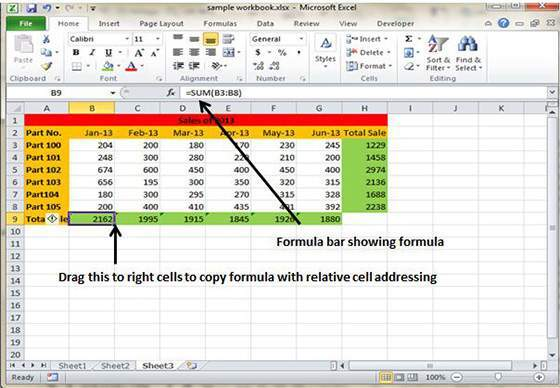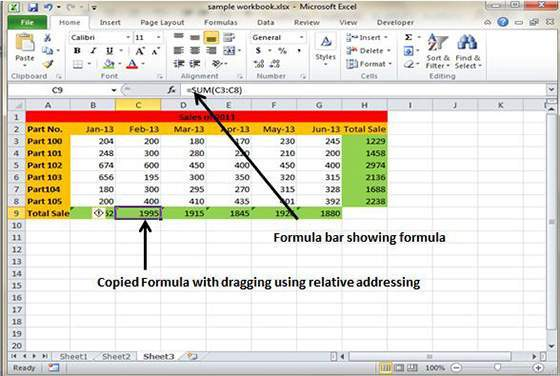# How to copy formulas in MS Excel 2010

Copying formulas is among the most common task performed by the users in the worksheet. Most of the formulas doesn’t use constant values rather the cell references and Excel is smart at copying the original formulas to every single place where users requires it.

MS Excel has the ability to automatically adjust the cell references in the original formula in order to ensure it works well in the copied cells. This is done by system called relative cell addresses which take the relative cell address in the original formula and changes the cell addresses to suit new column position and cell references.

Here is a fine example which will show how it works.

The ‘formula bar’ here shows the original formula which is used to calculate the sum for the rows from 3 to 8 in the 9th row.Now drag the formula to the remaining columns and it will get copied instantly with the subsequent changes in the relative cell references. After dragging the formula will show different and accurate column and cell references like this:

• Column C: =SUM(C3:C8)
• Column D: =SUM(D3:D8)
• Column E: =SUM(E3:E8)
• Column F: =SUM(F3:F8)
• Column G: =SUM(G3:G8)